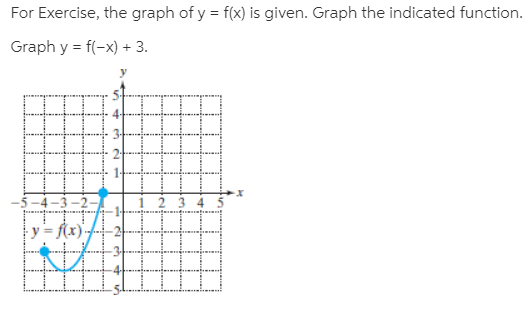# For Exercise, the graph of y = f(x) is given. Graph the indicated function. Graph y = f(-x) + 3. y = f(x)'---

Questionhelp_outlineImage TranscriptioncloseFor Exercise, the graph of y = f(x) is given. Graph the indicated function. Graph y = f(-x) + 3. y = f(x)'--- fullscreen